Introduction to Mixed Modeling Procedures

# Overview: Mixed Modeling Procedures

A mixed model is a model that contains fixed and random effects. Since all statistical models contain some stochastic component and many models contain a residual error term, the preceding sentence deserves some clarification. The classical linear model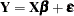contains the parameters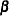and the random vector. The vectoris a vector of fixed-effects parameters; its elements are unknown constants to be estimated from the data. A mixed model in the narrow sense also contains random effects, which are unobservable random variables. If the vector of random effects is denoted by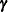, then a linear mixed model can be written as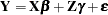In a broader sense, mixed modeling and mixed model software is applied to special cases and generalizations of this model. For example, a purely random effects model,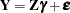, or a correlated-error model,, is subsumed by mixed modeling methodology.

Over the last few decades virtually every form of classical statistical model has been enhanced to accommodate random effecs. The linear model has been extended to the linear mixed model, generalized linear models have been extended to generalized linear mixed models, and so on. In parallel with this trend, SAS/STAT software offers a number of classical and contemporary mixed modeling tools. The aim of this chapter is to provide a brief introduction and comparison of the procedures for mixed model analysis (in the broad sense) in SAS/STAT software. The theory and application of mixed models are discussed at length in many monographs, including Milliken and Johnson (1992), Diggle, Liang, and Zeger (1994), Davidian and Giltinan (1995), Verbeke and Molenberghs (1997, 2000), Vonesh and Chinchilli (1997), Demidenko (2004), Molenberghs and Verbeke (2005), and Littell et al. (2006).

The following procedures in SAS/STAT software can perform mixed and random effects analysis to various degrees:

GLM

is primarily a tool for fitting linear models by least squares. The GLM procedure has some capabilities for including random effects in a statistical model and for performing statistical tests in mixed models. Repeated measures analysis is also possible with the GLM procedure, assuming unstructured covariance modeling. Estimation methods for covariance parameters in PROC GLM are based on the method of moments, and a portion of its output applies only to the fixed-effects model.

GLIMMIX

fits generalized linear mixed models by likelihood-based techniques. As in the MIXED procedure, covariance structures are modeled parametrically. The GLIMMIX procedure also has built-in capabilities for mixed model smoothing and joint modeling of heterocatanomic multivariate data.

HPMIXED

fits linear mixed models with simple covariance structures by sparse-matrix techniques. The experimental HPMIXED procedure is designed to handle large mixed model problems, such as the solution of mixed model equations with thousands of fixed-effects parameters and random-effects solutions.

LATTICE

computes the analysis of variance and analysis of simple covariance for data from an experiment with a lattice design. PROC LATTICE analyzes balanced square lattices, partially balanced square lattices, and some rectangular lattices. Analyses performed with the LATTICE procedure can also be performed as mixed models for complete or incomplete block designs with the MIXED procedure.

MIXED

performs mixed model analysis and repeated measures analysis by way of structured covariance models. The MIXED procedure estimates parameters by likelihood or moment-based techniques. You can compute mixed model diagnostics and influence analysis for observations and groups of observations. The default fitting method maximizes the restricted likelihood of the data under the assumption that the data are normally distributed and any missing data are missing at random. This general framework accommodates many common correlated-data methods, including variance component models and repeated measures analyses.

NESTED

performs analysis of variance and analysis of covariance for purely nested random-effects models. Because of its customized algorithms, PROC NESTED can be useful for large data sets with nested random effects.

NLMIXED

fits mixed models in which the fixed or random effects enter nonlinearly. The NLMIXED procedure requires that you specify components of your mixed model via programming statements. Some built-in distributions enable you to easily specify the conditional distribution of the data, given the random effects.

VARCOMP

estimates variance components for random or mixed models.

The focus in the remainder of this chapter is on procedures designed for random effects and mixed model analysis: the GLIMMIX, HPMIXED, MIXED, NESTED, NLMIXED, and VARCOMP procedures. The important distinction between fixed and random effects in statistical models is addressed in the section Fixed, Random, and Mixed Models, in Chapter 3, Introduction to Statistical Modeling with SAS/STAT Software.Previous Page | Next Page | Top of Page